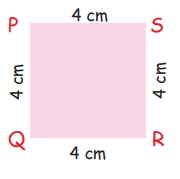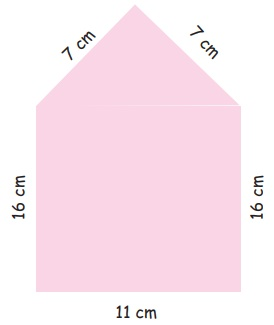Home | | Maths 5th Std | Perimeter of the rectangle and square

# Perimeter of the rectangle and square

Formula for the perimeter of a rectangle and square. Examples, Solution on perimeter of a rectangle and square.

UNIT−1

GEOMETRYPerimeter and Area

Perimeter of the rectangle and square

Situation

Raju is a class 5 student of a Panchayat union middle school. Raju's head master wanted to fence the school as there is disturbance caused by the tresspassers. Hence the headmaster decided to fence the school.The headmaster asked Raju and his classmates to measure the length of the boundary of the school premises, so as to buy the required fencing wire.

Raju and his friends measured the length of each side and added them to find the total length.

Length of the boundary = sum of the length of each side

Here the length of the boundary is called as perimeter,

Hence, the sum of the length of all the sides of a closed area is called its perimeter

Perimeter is used in framing a photo, for fencing a vacant land, etc..,

Activity 1

Write the perimeter of each figure:1.Perimeter of rectangle

P = (2×1) + (2×b)

= (2×6) + (2×5)

= 12 + 10

P = 22cm

2.Perimeter of square

P = 4 × a

= 4×4

P = 16cm

3.Perimeter of triangle

P= a+b+c

= 3+7+5

P = 15cm

4.Perimeter

P = 9+6+3+4+3+4+3+6

P= 38 cm

5.Perimeter

P = 10+5+5+10+5+5

P = 40 cm

6.Perimeter

P = 11+16+7+7+16

P = 57 cm

Formula for the perimeter of a rectangle:Perimeter = length + breadth + length + breadth (opposite sides of a rectangle are equal)

So, the perimeter of a rectangle = twice the length + twice the breadth

Perimeter of a rectangle = (2 × length) + (2 × breadth)

Example 1.1

The length of the rectangle below is 5cm and its breadth is 2cm. Find its perimeter.Solution

Perimeter of rectangle PQRS = (2 × length) + (2 × breadth)

= (2 × 5) + (2 × 2)

= 10+4

= 14

Therefore, the perimeter of the rectangle is 14 cm.

Formula for the perimeter of a squareThe lengths of all the sides of a square are equal.

Therefore, the perimeter of a square = four time the length of one of its sides

Perimeter of a square = 4 × (the length of one side)

Example 1.2

The length of one side of a square is 7cm. Find its perimeter.

Solution

The perimeter of a square is four times the length of one side.

Perimeter of a square = 4 × (length of one side)

= 4 × 7

= 28

Therefore, the perimeter of the square is 28 cm

Example 1.3

The length of a rectangular park is 60 cm, while its width is 50 cm. What is its perimeter?

Solution

Perimeter of the rectangle = (2 × length) + (2 × breadth)

= (2 × 60) + (2 × 50)

= 120 + 100

= 220

Therefore, the perimeter of the rectangular park is 220 cm.

Example 1.4

How much wire will be needed to put a triple fence around a square plot with side 20m? What will be the total cost of the wire at ₹ 50 per metre?

Solution

To put a single fence around the square plot, we need to find its perimeter

Perimeter of a square = 4 × length of one side = 4 × 20 = 80

The perimeter of the square plot is 80 metres. Since the fence is to be a triple fence we must triple the perimeter

80 × 3 = 240 m of wire will be needed to fence the square plot.

Now let us find out how much the wire will cost. One metre of wire costs ₹ 50

Therefore the cost of 240 m of wire will be 240 × 50 = 12,000

The total cost of wire for putting a triple fence around the plot will be ₹ 12,000

Try thisIf a square of side 1 cm is cut out of the corner of a larger square with side 4 cm (See the figure). What will be the perimeter of the remaining shape?

Perimeter of square P = 4 × a

= 4×4

= 16cm

Perimeter of cut out square P = 4 × a

= 4 × 1

= 4cm

Perimeter of the remaining shape = 16−4

= 12 cm

Tags : Geometry | Term 3 Chapter 1 | 5th Maths , 5th Maths : Term 3 Unit 1 : Geometry
Study Material, Lecturing Notes, Assignment, Reference, Wiki description explanation, brief detail
5th Maths : Term 3 Unit 1 : Geometry : Perimeter of the rectangle and square | Geometry | Term 3 Chapter 1 | 5th Maths